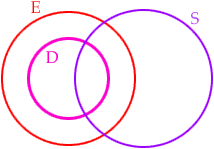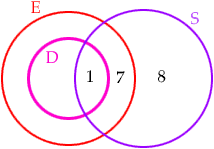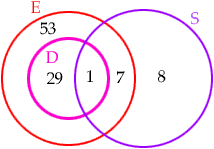Name: John and Norman Question: All Derfs are Enajs. One-third of all Enajs are Derfs. Half of all Sivads are Enajs. One Sivad is a Derf. Eight Sivads are Enajs. The number of Enajs is 90. How many Enajs are neither Derf nor Sivad? Hi, I would do this with a Venn diagram. Draw three circles, one for the Derfs one for the Enajs and one for the Sivads. Label them D, E and S. Since "All Derfs are Enajs" the D circle is inside the E circleNow look for clues that have specific numbers. "One Sivad is a Derf." tells you that you can put a 1 in the intersection of the S circle and the D circle. "Eight Sivads are Enajs." says that there are 8 in the intersection of the S and E circles. You already have 1 in that intersection so you need 7 more. "Half of all Sivads are Enajs." tells you that there are 8 inside S but outside E. Thus far you have:Finally "The number of Enajs is 90." and "One-third of all Enajs are Derfs." implies that there are 30 inside D and hence 29 inside D but outside S. Hence to have 90 inside E there must be 58 in the remaining piece of E.So, how many Enajs are neither Derf nor Sivad? Cheers, Penny Go to Math Central